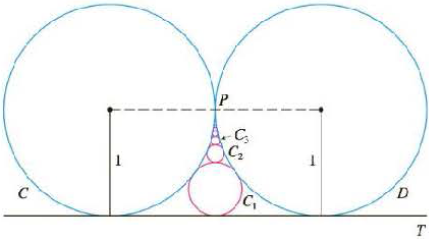Chapter 11.2, Problem 79E

Chapter
Section
Textbook Problem

# The figure shows two circles C and D of radius 1 that touch at P. The line T is a common tangent line; C1 is the circle that touches C, D, and T; C2 is the circle that touches C, D, and C1; C3 is the circle that touches C, D, and C2. This procedure can be continued indefinitely and produces an infinite sequence of circles {Cn}. Find an expression for the diameter of Cn and thus provide another geometric demonstration of Example 8.To determine

To find: The expression for the diameter cn .

Explanation

Given:

The two circles C and D of radius 1 touch at P.

Calculation:

Assume that the center of the circle D is R and the center of the circle C1 is A and the triangle PRA is a right triangle

By the Pythagoras theorem,

(AP)2+(PR)2=(AR)2(1d12)2+12=(1+d12)21d1+d124+1=1+d1+d124

Simplify further,

1d1+=1+d22d2=1d2=12

Assume that the center of the circle D is R and the center of the circle C2 is B and the triangle PRB is a right triangle

By the Pythagoras theorem,

(PB)2+(PR)2=(BR)2(1(d1d22))2+12=(1+d22)2(1(12+d22))2+1=(1+d22)212(12+d22)+(12+d22)2=1+d2+d224

That is, d2=16 .

Assume that the center of the circle D is R and the center of the circle C3 is C and the triangle PRB is a right triangle

By the Pythagoras theorem

(PC)2+(PR)2=(CR)2(1(d1+d2+d32))2+12=(1+d32)2(1(12+16+d32))2+1=(1+d32)2(1(23+d32))2+1=(1+d32)2

That is, d3=112 .

The diameters d1,d2 and d3 can be expressed as follows,

d1=11×2d2=12×3d3=13×4

Therefore, it can be conclude that dn=1n(n+1)

### Still sussing out bartleby?

Check out a sample textbook solution.

See a sample solution

#### The Solution to Your Study Problems

Bartleby provides explanations to thousands of textbook problems written by our experts, many with advanced degrees!

Get Started